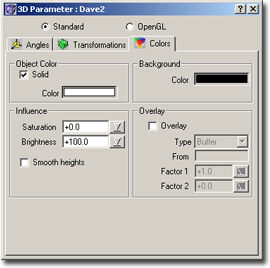### 3D Parameter Window – Colors TabFig.: 3D Parameters – Colors Tab

• Object Color
If Solid is checked, then the color defined below the checkbox defines the color used to draw the image. The intensity of this color is automatically replaced by the light intensity for a pixel. The effect is, that the whole image appears to be in shades of this color.
If Solid is not checked, then the original colors of the fractal are used. The intensity component of each pixel is replaced by the light intensity, which shines onto that area.
• Background
Here you can define the background color to use for the 3D view
• Influence
Here you can define the influence which the light intensity has on the color of a pixel to draw. The light intensity can have influence on the saturation and on the brightness of the color.
0 means Does not have any influence
100 means Does replace that component

An example: a pixel is examined, the height and the color is calculated. After that the color is transformed into the HSB color space. The intensity of the light, which falls onto this pixel, is calculated. Now how does the light affect the color?

Assume: Saturation=0, Brightness=100

Then the saturation component is left unchanged, the brightness component directly gets the light intensity.

• Smooth heights
This is perhaps the most useful option of all 3D options: If selected, then the heights are automatically smoothed. This greatly improves the quality of the resulting 3D image. Without selecting this box the heights are directly taken from the buffer, just as they were calculated. But this leads to steep valeys or mountains, looking totally unnatural (and ugly).

Overlay

Using this feature you can overlay the current fractal with another fractal, for example you could overlay the mandelbrot set with a plasma fractal, thus the 3D image of the mandelbrot sets gets some texture.

To enable overlaying you must place a check mark into the checkbox. Then you must enter the name of the other fractal, from which the information for the overlaying should be taken, i.e. the name of the fractal which should be ‘added’ to this fractal. It must specify a calculated fractal in the same folder.
There exist 4 modes for overlaying:

• Buffer
Here the buffer values of the two fractals are taken and added. After that the height function is applied. Here the effect of the other (added) fractal depends on the height transformation function: Suppose you have the Mandelbrot set and your height function maps the whole ‘lake’ to one single height, a plateau. If you now add another fractal to this, then the lake will stay the same, you won’t notice that another fractal is added, because the height transformation function maps all values above, say: 40, to one single height. On the other hand, the ascending walls of the Mandelbrot mountain get distorted, because there a value is added and the height transformation function is sensible in those intervals, where small buffer values exist.
• Height
Here the height function is applied to each of the two fractals. The resulting heights are then added and drawn directly.
In this mode the whole fractal is distorted, according to the height transformation function.
• Intensity (Buffer)
Here the intensity of the color of the other fractal is added to the buffer value of the original fractal. Then the height function is applied
• Intensity (Height)
This mode now takes the intensity of the color, applies the height function to each of the two fractals and then the two heights are added.

Note: The previous two modes allow you to load an arbitrary image and overlay a fractal with that image. Although the loaded image is not a fractal, it has some data (i.e. image data like intensity). You then can overlay a fractal with that image. Imagine you have an image which shown the New York skyline. You load it into ChaosPro and then you can overlay a Julia set with the New York skyline.